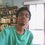# Triangle-Area Problem

Through an arbitrary point lying inside a triangle,3 straight lines parallel to its sides are drawn. These lines divide the triangle into six parts ,3 of which are triangles. If the areas of these triangles are A,B,C,then what is the area of the initially given triangle?(obviously area is to found in terms of A,B and C)Note by Biswaroop Roy
6 years, 9 months ago

This discussion board is a place to discuss our Daily Challenges and the math and science related to those challenges. Explanations are more than just a solution — they should explain the steps and thinking strategies that you used to obtain the solution. Comments should further the discussion of math and science.

When posting on Brilliant:

• Use the emojis to react to an explanation, whether you're congratulating a job well done , or just really confused .
• Ask specific questions about the challenge or the steps in somebody's explanation. Well-posed questions can add a lot to the discussion, but posting "I don't understand!" doesn't help anyone.
• Try to contribute something new to the discussion, whether it is an extension, generalization or other idea related to the challenge.

MarkdownAppears as
*italics* or _italics_ italics
**bold** or __bold__ bold
- bulleted- list
• bulleted
• list
1. numbered2. list
1. numbered
2. list
Note: you must add a full line of space before and after lists for them to show up correctly
paragraph 1paragraph 2

paragraph 1

paragraph 2

[example link](https://brilliant.org)example link
> This is a quote
This is a quote
    # I indented these lines
# 4 spaces, and now they show
# up as a code block.

print "hello world"
# I indented these lines
# 4 spaces, and now they show
# up as a code block.

print "hello world"
MathAppears as
Remember to wrap math in $$ ... $$ or $ ... $ to ensure proper formatting.
2 \times 3 $2 \times 3$
2^{34} $2^{34}$
a_{i-1} $a_{i-1}$
\frac{2}{3} $\frac{2}{3}$
\sqrt{2} $\sqrt{2}$
\sum_{i=1}^3 $\sum_{i=1}^3$
\sin \theta $\sin \theta$
\boxed{123} $\boxed{123}$

Sort by:

Some one please try this one. I am working on it for quite some time now,and I would be glad if some one solves it.

- 6 years, 9 months ago

If X is the area of the triangle, then sqrt(A)/sqrt(X) is the ratio of the corresponding sides. Adding ratios of the corresponding sides together gives 1 (you can easily see this from a picture), so sqrt(A)/sqrt(X) + sqrt(B)/sqrt(X) + sqrt(C)/sqrt(X) = 1.

- 6 years, 9 months ago

The answer that I got was 3(A+B+C) because think about it. The way this question is posed, it must hold true for all triangles. If we let the triangle be an equilateral triangle, and let the intersection of these three lines be the center (remember, this is to make things easier), then we produce 3 rhombi and 3 triangles (like a hazardous sign). If we split the rhombi into equilateral triangles, you'll notice that we have 9 equal equilateral triangles, 3 of which are A, B, and C. This means that there are 3 times their sum. If you want to get down and dirty with the math, use Heron's formula and try messing around with that. Hope this helps!

- 6 years, 9 months ago

Answer is not 3(A+B+C). Originally the question had 4 options And obviously an option was 3(A+B+C) Needless to say I thought the answer was that.Surprising the answer turned out to be (sq.root(A)+sq.root(B)+sq.root(C))^2. I still haven't got this answer.And I am still working on it. You might notice that this answer also holds true for symmetric figures like equilateral triangle.

- 6 years, 9 months ago

Hmm... Nevermind then...

- 6 years, 9 months ago

Hint: Similar Triangles. Use this to find the areas of the 'medium triangles' and hence the parallelograms.

Staff - 6 years, 9 months ago

Thanks.

- 6 years, 8 months ago

The issue with assuming a specific case, is that your answer only works for that specific case, and you do not know what happens in the other cases. For example, we could get the same value using functions like $\frac{ A^2 + B^2 + C^2 } { A+B+C}$ or $\frac{ 9ABC} { AB + BC + CA }$.

You could do some further checks by taking other points, or looking at other triangles. For example, if you consider the point to be at a vertex, then $3(A+B+C)$ is clearly the wrong answer.

Staff - 6 years, 9 months ago